# Trigonometric Functions

 [ LiB ]

You probably learned some trigonometric functions in high school. The functions that you will see here are probably the most useful and common ones, and you may be familiar with them already. Remember that this won't be another boring math lesson but a cool way to put these once seemingly useless functions to good use.

#### The Sine

The sine of an angle is calculated as the opposite side of the angle divided into the hypotenuse of the right triangle. See Figure 11.9 for the visual.

##### Figure 11.9. Defining the sine of an angle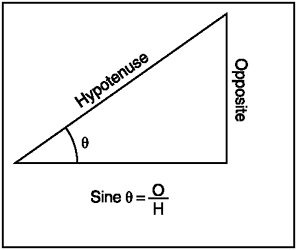You also might have learned other ways to represent the sine's values. Have you seen sine graphed as in Figure 11.10?

##### Figure 11.10. The sine graph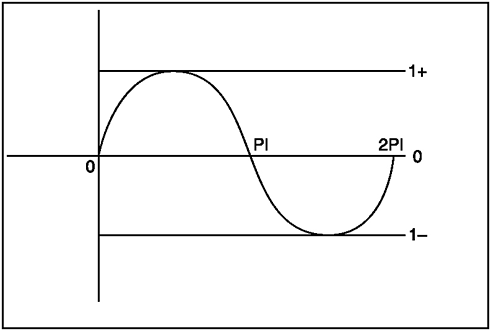Looks a little bit more interesting doesn't it? Did you know that sine is used in many games , and that I just happened to write up an example of how it can be used? Before we go on, notice how its values only output from 1 to 1; this allows you to scale these values to get whatever other values you want.

Check out demo GDA_PROG11.3.fla and see how I made the projectile just move as if the sine gods had ordered it so. Figure 11.11 shows the projectile that I used for this motion.

##### Figure 11.11. A sine projectile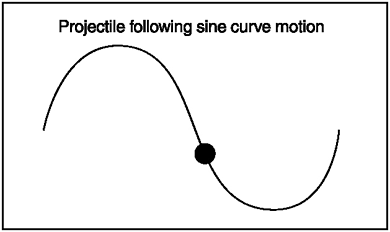Check out the SWF and then take a look at the listing.

` // Game Development with ActionScript // Lewis Moronta (c) 2003 // This program demonstrates how // to use Math.sin methods. degrees = 0; onEnterFrame = function () {   // Manipulate degrees   degrees+=10;   // Make sure it doesn't go overboard   if (degrees > 360)     degrees = 0;   // Convert to radians   var radians = Math.PI/180 * degrees;   // This stores -1 to 1   var sineY = Math.sin(radians);   // Move the projectile from 100 to 300   thing._y = sineY * 100 + 200   // Now that we got him moving up and down   // according to the power of the sine,   // let's move him across the screen =)   thing._x += 10;   if (thing._x > 550)     thing._x = 0; }; `

If the sine function didn't exist, you could probably pull the same thing with a few more if statements, but to tell you the truth, it wouldn't be as smooth. The sine function actually has really cool slow ins and outs at its peaks.

The first global variable that I set up was degrees . I used this variable to increment itself to 359 then loop back to 0. Most of the code is in the onEnterFrame handler that I needed, so I can move the object on every frame entry.

` degrees = 0; onEnterFrame = function () {  // Manipulate degrees degrees+=10; // Make sure it doesn't go overboard if (degrees > 360)   degrees = 0; `

In the previous section you learned how to convert degrees to radians because radians are the only values that sine accepts. This is why I used this formula again:

` // Convert to radians var radians = Math.PI/180 * degrees; `

For easier storage, and for convenience, I wrote the following line, one of the most important in this demo:

` // This stores -1 to 1 var sineY = Math.sin(radians); `

Once I acquired my value from the function, I know that I have an almost-predictable value that's between 1 and 1, so what I did was scale this range to something that the human eye can see. I scaled it to move from 100 to 300 on the Y-axis.

` // Move the projectile from 100 to 300   thing._y = sineY * 100 + 200 `

NOTE

NOTE

This works because when sine is 1 (and multiplied by 100) it will yield 100, so by adding 200 to it, the result will yield 100 (our y value). When sine is 1 (and multiplied by 100) it will yield 100, so by adding 200 to it, it will make our y value 300.

As this will happen on every frame, you will have a ball smoothly bouncing up and down. I wanted the Movie Clip to scroll so you can visually see the sine within this picture so I added the following line.

` thing._x += 10; `

And of course, I made it loop so you can continue to be mesmerized by the mathematical visual.

` if (thing._x > 550)     thing._x = 0; `

Is it just me, or does that projectile look like a good enemy for that game you're planning?

#### The Cosine

The next trig function we're going to discuss is similar to sine, and has an aptly similar namecosine. See Figure 11.12 for a visual explanation of how cosine is defined.

##### Figure 11.12. The cosine of an angle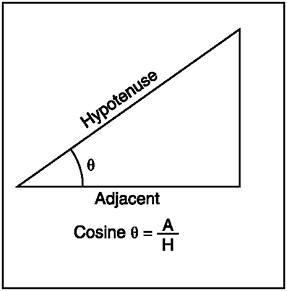Figure 11.12 tells you that the cosine of an angle is the adjacent side divided by the hypotenuse. How would this look graphically? See Figure 11.13.

##### Figure 11.13. The cosine graph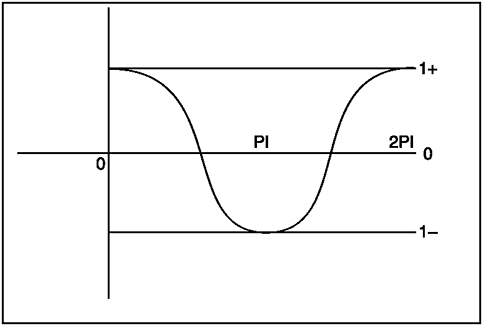As you examine the cosine graph, you'll note that it's very similar to the sine graph except that it's shifted to the right by 90 degrees (or Pi/2 for you math freaks). If you were to examine GDA_PROG11.4.fla, you would notice that the only difference from the sine version of the demo is that the projectile is receiving its values from the cosine function instead of the sine function. This causes the curve to start at a different point, but the curve itself is identical.

Let's check out the quick changes I made within this program.

` // Game Development with ActionScript // Lewis Moronta (c) 2003 // This program demonstrates how // to use Math.sin methods. degrees = 0; onEnterFrame = function () {   // Manipulate degrees   degrees+=10;   // Make sure it doesn't go overboard   if (degrees > 360)   degrees = 0;   // Convert to radians   var radians = Math.PI/180 * degrees;   // This stores -1 to 1    var cosineY = Math.cos(radians);   // Move the projectile from 100 to 300   thing._y = cosineY * 100 + 200   // Now that we got him moving up and down   // according to the power of the sine,   // let's move him across the screen =)   thing._x += 10;   if (thing._x > 550)     thing._x = 0; }; `

If you play the SWF movie, you'll notice that not much has changed except that the code makes the projectile move on a different (shifted) curve. All I did was change this function from sine to cosine.

NOTE

NOTE

This is a very basic introduction to the trigonometric functions. If you feel you need more, see the Math section in your local bookstore.To be honest with you, you already are equipped enough to build cool demos if you sit down with what you have. Don't let this information hold you back from learning more, though. Ask Andr LaMothehe'll tell you that you can't ever intake too much math.

#### The Tangent

The tangent function is a combination of the last two. The tangent of an angle is the sine of that angle divided by the cosine of that same angle. What does this mean? It means that the tangent of an angle can also be the opposite side of that angle divided by its adjacent side.

See Figure 11.14 for a mathematical definition of tangent.

##### Figure 11.14. The tangent of an angle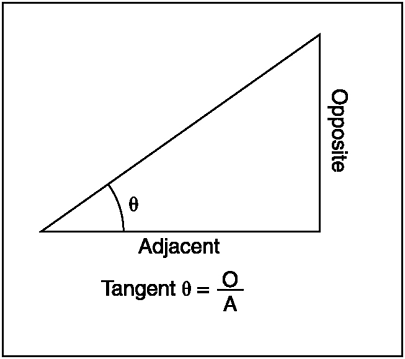The graph of tangent has many asymptotes, and it would be useless for me to show you the graph without telling you what an asymptote is. For definition purposes, an asymptote is a point on a graph where there is an unattainable value. The value can either be negative or positive, and the graph bends towards that direction. Observe Figure 11.15.

##### Figure 11.15. The tangent graph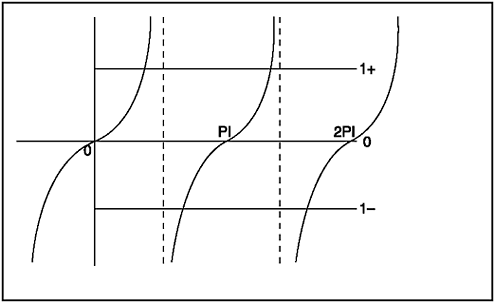See the dashed lines? As the curve gets closer to the dashed lines, it also gets closer to an unattainable positionevery vertical line in this graph is an asymptote.

 [ LiB ]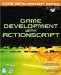Game Development with ActionScript
ISBN: 1592001106
EAN: 2147483647
Year: 2004
Pages: 162
Authors: Lewis Moronta